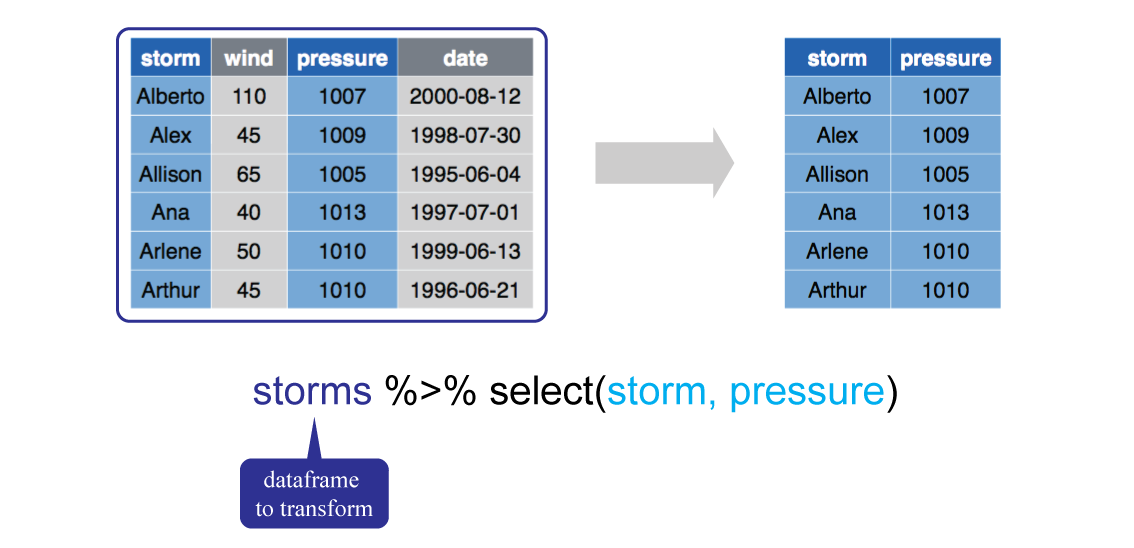August 6, 2016

because…

- Garrett Grolemund

What to Remember from this Section

dplyr is a package that transforms and manipulates data

select(): select variables of concern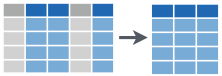filter(): filter values based on conditions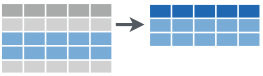group_by(): group data by categorical levels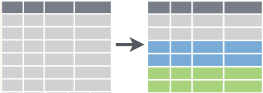summarise(): change unit of analysis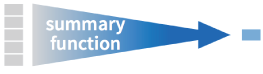arrange(): order the data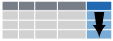What to Remember from this Section

dplyr is a package that transforms and manipulates data

mutate(): create new variables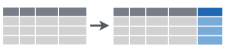join(): combine separate data sets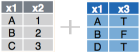R package used…

install.packages("dplyr")

library(dplyr)

data used…

install.packages("EDAWR")

library(EDAWR)

Data sets: storms, tb, pollution, iris, a, b

%>% operator…

learn it, love it, leverage it

filter(data, variable == numeric_value)

or

data %>% filter(variable == numeric_value)

%>% operator…

learn it, love it, leverage it

arrange(
summarize(
filter(data, variable == "numeric_value"),
Total = sum(variable)
),
desc(Total)
)
a <- filter(data, variable == "numeric_value")
b <- summarise(a, Total = sum(variable))
c <- arrange(b, desc(Total))
data %>%
14     filter(variable == "value") %>%
summarise(Total = sum(variable)) %>%
arrange(desc(Total))
Same results but the %>% operator is more efficient and legible

select( )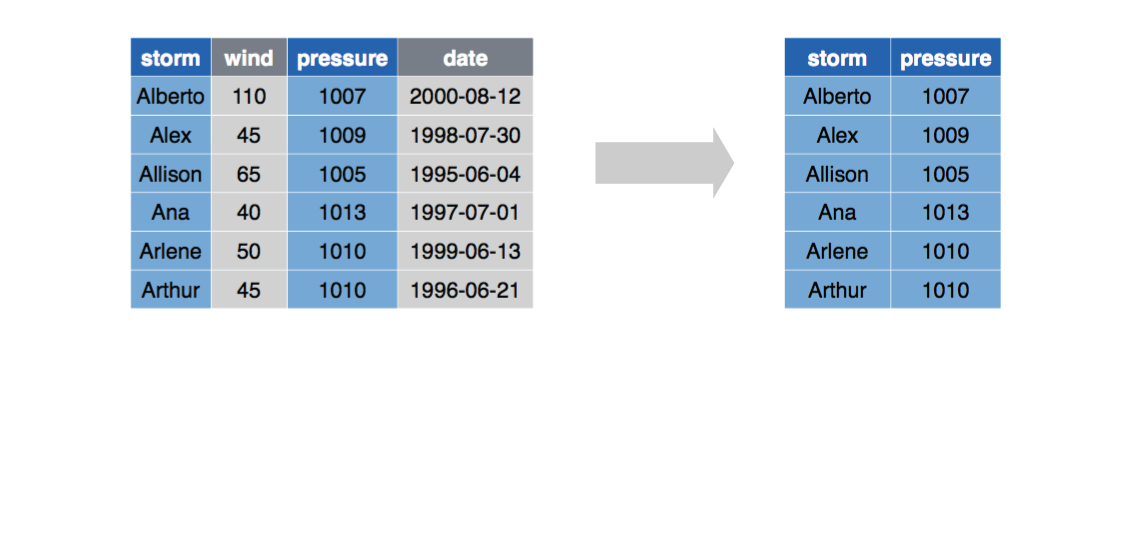select( )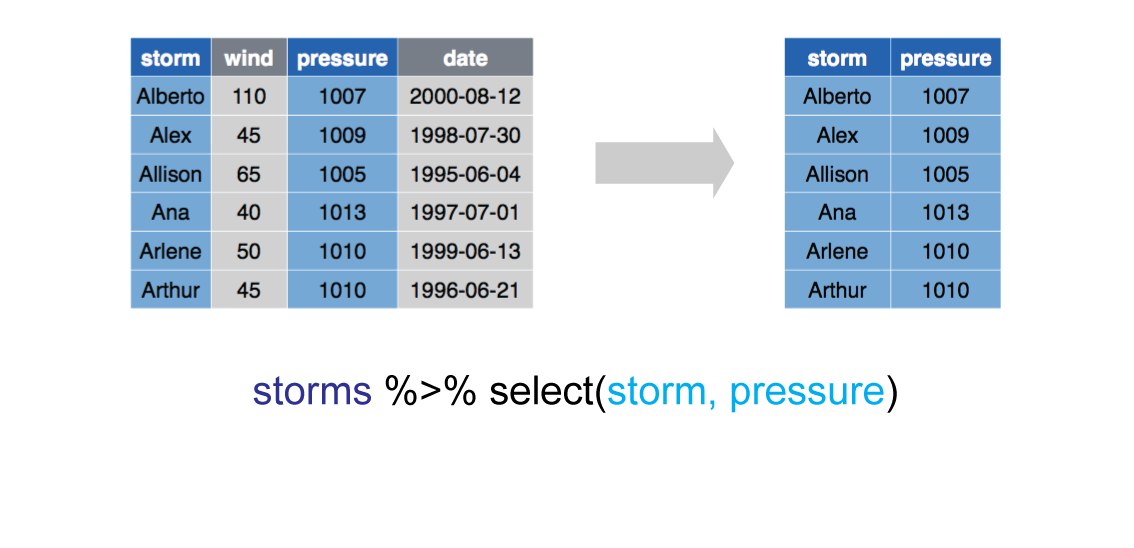select( )